Download Presentation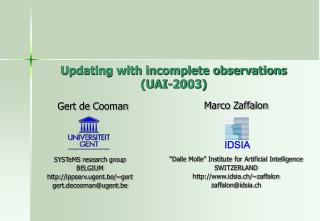Updating with incomplete observations (UAI-2003)

# Updating with incomplete observations (UAI-2003) - PowerPoint PPT Presentation

IDSIA. Updating with incomplete observations (UAI-2003). Gert de Cooman. Marco Zaffalon. “Dalle Molle” Institute for Artificial Intelligence SWITZERLAND http://www.idsia.ch/~zaffalon zaffalon@idsia.ch. SYSTeMS research group BELGIUM http://ippserv.ugent.be/~gert gert.decooman@ugent.be.I am the owner, or an agent authorized to act on behalf of the owner, of the copyrighted work described.
Download Presentation## Updating with incomplete observations (UAI-2003)

An Image/Link below is provided (as is) to download presentation

Download Policy: Content on the Website is provided to you AS IS for your information and personal use and may not be sold / licensed / shared on other websites without getting consent from its author.While downloading, if for some reason you are not able to download a presentation, the publisher may have deleted the file from their server.

- - - - - - - - - - - - - - - - - - - - - - - - - - E N D - - - - - - - - - - - - - - - - - - - - - - - - - -
Presentation Transcript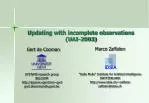IDSIA

### Updating with incomplete observations(UAI-2003)

Gert de Cooman

Marco Zaffalon

“Dalle Molle” Institute for Artificial Intelligence

SWITZERLAND

http://www.idsia.ch/~zaffalon

zaffalon@idsia.ch

SYSTeMS research group

BELGIUM

http://ippserv.ugent.be/~gert

gert.decooman@ugent.be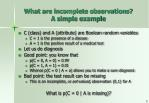What are incomplete observations?A simple example
• C (class) and A (attribute) are Boolean random variables
• C = 1 is the presence of a disease
• A = 1 is the positive result of a medical test
• Let us do diagnosis
• Good point: you know that
• p(C = 0, A = 0) = 0.99
• p(C = 1, A = 1) = 0.01
• Whence p(C = 0 | A = a) allows you to make a sure diagnosis
• Bad point: the test result can be missing
• This is an incomplete, or set-valued, observation {0,1} for A

What is p(C = 0 | A is missing)?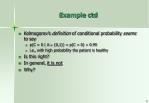Example ctd
• Kolmogorov’s definition of conditional probability seems to say
• p(C = 0 | A  {0,1}) = p(C = 0) = 0.99
• i.e., with high probability the patient is healthy
• Is this right?
• In general, it is not
• Why?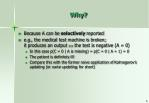Why?
• Because A can be selectively reported
• e.g., the medical test machine is broken;it produces an output  the test is negative (A = 0)
• In this case p(C = 0 | A is missing) = p(C = 0 | A = 1) = 0
• The patient is definitely ill!
• Compare this with the former naive application ofKolmogorov’s updating (or naive updating, for short)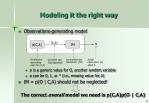(c,a)

o

IM

p(C,A)

Incompleteness Mechanism (IM)

Actual observation (o) about A

Distribution generating pairs for (C,A)

Complete pair (not observed)

Modeling it the right way
• Observations-generating model
• o is a generic value for O, another random variable
• o can be 0, 1, or * (i.e., missing value for A)
• IM = p(O | C,A) should not be neglected!

The correct overall model we need is p(C,A)p(O | C,A)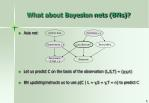(V)isit to Asia

(S)moking = y

(T)uberculosis = n

Lung (C)ancer?

Bronc(H)itis

Abnorma(L) X-rays = y

(D)yspnea

What about Bayesian nets (BNs)?
• Asia net
• Let us predict C on the basis of the observation (L,S,T) = (y,y,n)
• BN updating instructs us to use p(C | L = y,S = y,T = n) to predict C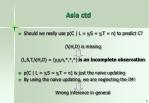Asia ctd
• Should we really use p(C | L = y,S = y,T = n) to predict C?

(V,H,D) is missing

(L,S,T,V,H,D) = (y,y,n,*,*,*) is an incomplete observation

• p(C | L = y,S = y,T = n) is just the naive updating
• By using the naive updating, we are neglecting the IM!

Wrong inference in general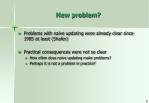New problem?
• Problems with naive updating were already clear since 1985 at least (Shafer)
• Practical consequences were not so clear
• How often does naive updating make problems?
• Perhaps it is not a problem in practice?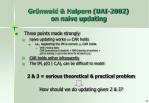Grünwald & Halpern (UAI-2002) on naive updating
• Three points made strongly
• naive updating works  CAR holds
• i.e., neglecting the IM is correct  CAR holds
• With missing data:CAR (coarsening at random) = MAR (missing at random) =p(A is missing | c,a) is the same for all pairs (c,a)
• CAR holds rather infrequently
• The IM, p(O | C,A), can be difficult to model

2 & 3 = serious theoretical & practical problem

How should we do updating given 2 & 3?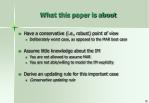What this paper is about
• Have a conservative (i.e., robust) point of view
• Deliberately worst case, as opposed to the MAR best case
• Assume little knowledge about the IM
• You are not allowed to assume MAR
• You are not able/willing to model the IM explicitly
• Derive an updating rule for this important case
• Conservative updating rule(c,a)

o

IM

p(C,A)

Unknown Incompleteness Mechanism

Actual observation (o) about A

Known prior distribution

Complete pair (not observed)

1st step: plug ignorance into your model
• Fact: the IM is unknown
• p(O{0,1,*} | C,A) = 1
• a constraint on p(O | C,A)
• i.e. any distribution p(O | C,A) is possible
• This is too conservative;to draw useful conclusionswe need a little less ignorance
• Consider the set of all p(O | C,A) s.t. p(O | C,A) = p(O | A)
• i.e., all the IMs which do not depend on what you want to predict
• Use this set of IMs jointly with prior information p(C,A)2nd step: derive the conservative updating
• Let E = evidence = observed variables, in state e
• Let R = remaining unobserved variables (except C)
• Formal derivation yields:
• All the values for R should be considered
• In particular, updating becomes:

Conservative Updating Rule(CUR)

minrRp(c | E = e,R = r) p(c | o)  maxrRp(c | E = e,R = r)(V)isit to Asia

(S)moking = y

(T)uberculosis = n

Lung (C)ancer?

Bronc(H)itis

Abnorma(L) X-rays = y

(D)yspnea

CUR & Bayesian nets
• Evidence: (L,S,T) = (y,y,n)
• What is your posterior confidence on C = y?
• Consider all the jointvalues of nodes in RTake min & max of p(C = y | L = y,S = y,T = n,v,h,d)

Posterior confidence  [0.42,0.71]

• Computational note: only Markov blanket matters!A few remarks
• The CUR…
• is based only on p(C,A), like the naive updating
• produces lower & upper probabilities
• can produce indecisionCUR & decision-making
• Decisions
• c’ dominates c’’ (c’,c’’ C) if for all r R ,

p(c’ | E = e, R = r) > p(c’’ | E = e, R = r)

• Indecision?
• It may happen that r’,r’’ R so that:

p(c’ | E = e, R = r’) > p(c’’ | E = e, R = r’)

and

p(c’ | E = e, R = r’’) < p(c’’ | E = e, R = r’’)

There is no evidence that you should prefer c’ to c’’ and vice versa

(= keep both)(V)isit to Asia

(S)moking = y

(T)uberculosis

Lung (C)ancer?

Bronc(H)itis

Abnorma(L) X-rays = y

(D)yspnea

Decision-making example
• Evidence: E = (L,S,T) = (y,y,n) = e
• What is your diagnosis for C?
• p(C = y | E = e, H = n, D = y) > p(C = n | E = e, H = n, D = y)
• p(C = y | E = e, H = n, D = n) < p(C = n | E = e, H = n, D = n)
• Both C = y and C = n are plausible
• Evidence:E = (L,S,T) = (y,y,y) = e
• C = n dominates C = y: “cancer” is ruled outAlgorithmic facts
• CUR  restrict attention to Markov blanket
• State enumeration still prohibitive in some cases
• e.g., naive Bayes
• Dominance test based on dynamic programming
• Linear in the number of children of class node C

However:

decision-making possible in linear time, by provided algorithm, even on some multiply connected nets!On the application side
• Important characteristics of present approach
• Robust approach, easy to implement
• Does not require changes in pre-existing BN knowledge bases
• based on p(C,A) only!
• Markov blanket  favors low computational complexity
• If you can write down the IM explicitly, your decisions/inferences will be contained in ours
• By-product for large networks
• Even when naive updating is OK, CUR can serve as a useful preprocessing phase
• Restricting attention to Markov blanket may produce strong enough inferences and decisionsWhat we did in the paper
• Theory of coherent lower previsions (imprecise probabilities)
• Coherence
• Equivalent to a large extent to sets of probability distributions
• Weaker assumptions
• CUR derived in quite a general frameworkConcluding notes
• There are cases when:
• IM is unknown/difficult to model
• MAR does not hold
• Serious theoretical and practical problem
• CUR applies
• Robust to the unknown IM
• Computationally easy decision-making with BNs
• CUR works with credal nets, too
• Same complexity
• Future: how to make stronger inferences and decisions
• Hybrid MAR/non-MAR modeling?